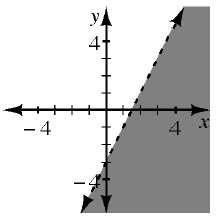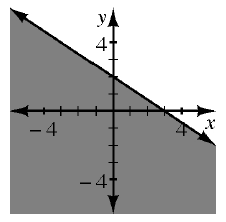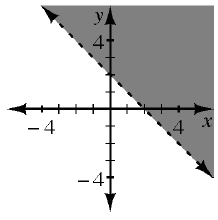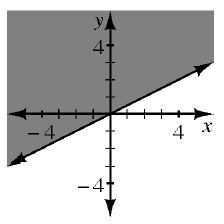### Home > CCAA8 > Chapter 9 Unit 10 > Lesson CCA: 9.4.1 > Problem9-94

9-94.

Match each graph below with the correct inequality.

1. $y>-x+2$

Which graph represents the line $y=-x+2$?

Graph 3

1. $y<2x-3$

Follow the steps in part (a).

Graph 1

1. $y\ge\frac{1}{2}x$

Follow the steps in part (a).

1. $y\le-\frac{2}{3}x+2$

Follow the steps in part (a).

1.1.1.1.